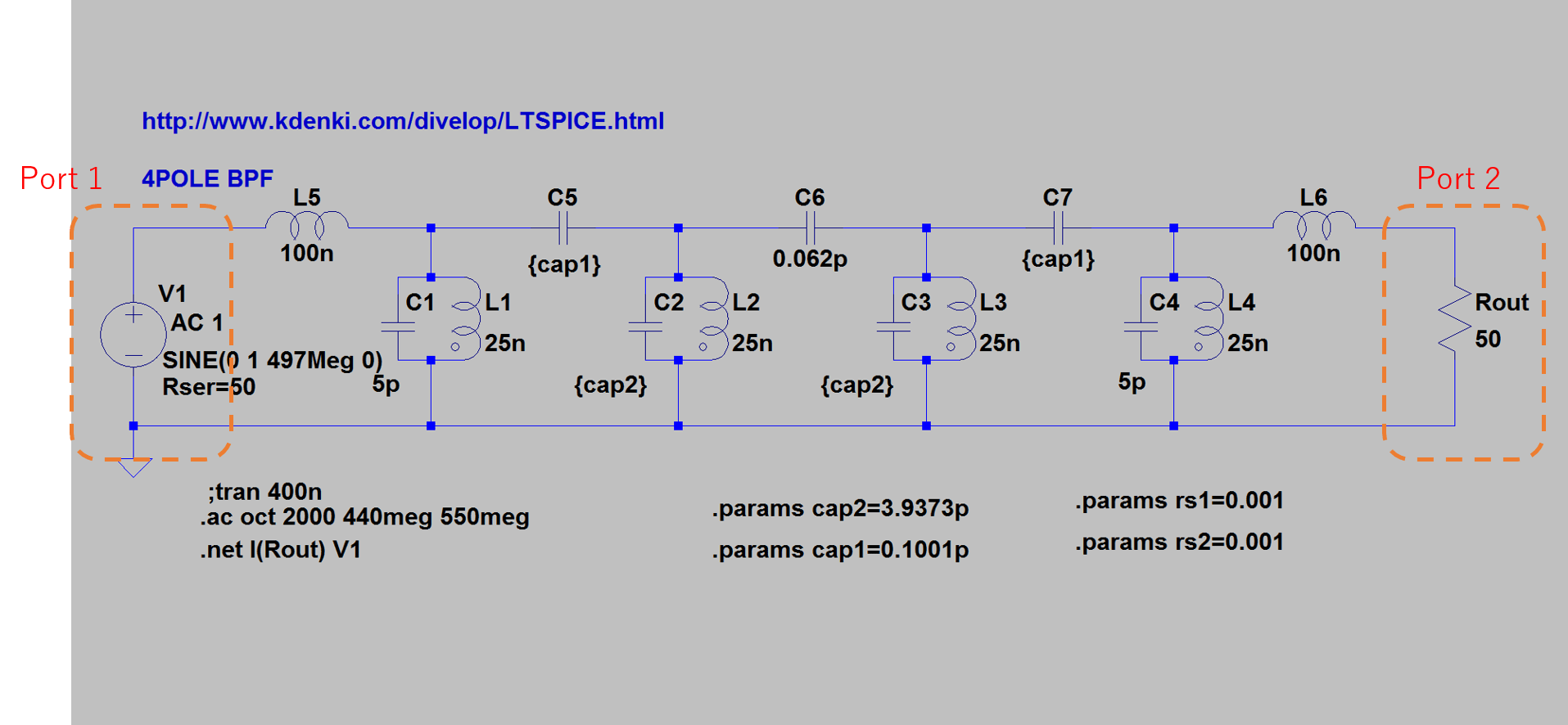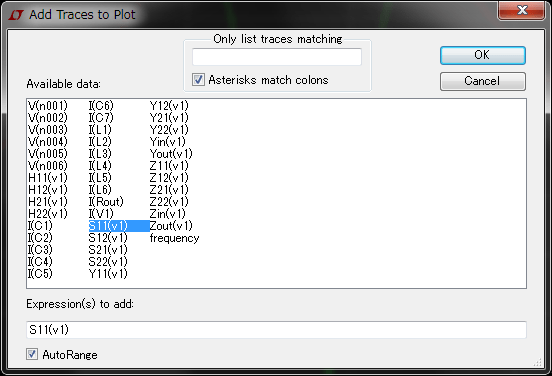The .net Spice function can be used to calculate two port parameters such as Z,Y,H and S parameters. Zin and Zout can also be calculated.

The circuit below shows a simple example. This is the bans pass filter (BPF).

Here is the LTspice file.

ltspice_sparameter1. Create an input port V1 with a series resistance. The series resistance will typically be 50 Ohm for S-parameters. This resistance will not influence the Z,Y,H parameters or Z in. It will influence the value of Zout. You can choose this value as 0 Ohm for an ideal source.

2. Create an output port with a load resistance Rout. The load resistance will typically be 50 Ohm for S-parameters. This resistance will not influence the Z,Y,H parameters or Zout. It will influence the value of Zin. You can choose this value very high if you don’t have a load.

3. Use the function .net I(Rout) V1 to be able to plot the two port parameters.4. Run the simulation. Then plot the parameters by using PlotSetting/AddTrace (or CTRL-A) while in the Plot menu.## 2件のコメント

1. […] S-parameters with LTSpice […]

2.Ali

Dear
I have a filter with its s-parameters data.
I want to put it in a circuit to simulate the circuit. (actually this filter is a part of a circuit in LTspice.)
How should I do it?

Thanks

このサイトはスパムを低減するために Akismet を使っています。コメントデータの処理方法の詳細はこちらをご覧ください